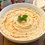# Squaring numbers mentally up to 125

I dont know if you guys already know about this, but i have a trick for squaring numbers from 0-125 but i have 2 different strategies,some work better than others sometimes.(4 if you didnt memorize squares of #s up to 25).

Note:the trick works for all real numbers but it is easily mentally computed over the range of integers from 0 to 125

Trick #1 -

for numbers more than 25 but less than 75.(this is because if the number is more than 75 you will have to do more calculations);

let the number be n ,the steps are as follows

-calculate n-25

-multiply it by 100

-calculate 50-n

-square it then add it to n-25

ex:${39}^{2}$

$\boxed{1}$ (39-25)*100=1400

$\boxed{2}$ ${11}^{2}$=121 so 1400+121=1521

Trick #2-

if the number is more than 75 but less than 125 we have this rule:

-calculate 100-n

-subtract your result from the original number then multiply it by 100

-square (100-n) then add it to the previous result

ex:${109}^{2}$

100-109=-9

100(109+9)=11800

adding ${9}^{2}$ we get 11881

These methods require you to know squares of numbers up to 25,

this is a trick just to help you if you forgot

for 2 digit numbers starting with 1:

-add the ones digit to the number

-multiply it by 10 ,then add the square of the ones digit

for 2 digit #s starting with 2:

-add the ones digit to the number

-multiply it by 20

-add the square of the ones digit

$ex:15\\ \\ 15+5=20\\ 20*10=200\quad \quad {5}^ {2}=25\quad \quad \quad \quad 200+25=225\\ \\ ex:23\\ 23+3=26\Rightarrow 2*260\Rightarrow 520+{3}^ {2}=529\\ \\ \\ \\ \$/extract_itex]Note by Hamza A 5 years, 4 months ago This discussion board is a place to discuss our Daily Challenges and the math and science related to those challenges. Explanations are more than just a solution — they should explain the steps and thinking strategies that you used to obtain the solution. Comments should further the discussion of math and science. When posting on Brilliant: • Use the emojis to react to an explanation, whether you're congratulating a job well done , or just really confused . • Ask specific questions about the challenge or the steps in somebody's explanation. Well-posed questions can add a lot to the discussion, but posting "I don't understand!" doesn't help anyone. • Try to contribute something new to the discussion, whether it is an extension, generalization or other idea related to the challenge. • Stay on topic — we're all here to learn more about math and science, not to hear about your favorite get-rich-quick scheme or current world events. MarkdownAppears as *italics* or _italics_ italics **bold** or __bold__ bold - bulleted- list • bulleted • list 1. numbered2. list 1. numbered 2. list Note: you must add a full line of space before and after lists for them to show up correctly paragraph 1paragraph 2 paragraph 1 paragraph 2 [example link](https://brilliant.org)example link > This is a quote This is a quote  # I indented these lines # 4 spaces, and now they show # up as a code block. print "hello world" # I indented these lines # 4 spaces, and now they show # up as a code block. print "hello world" MathAppears as Remember to wrap math in $$ ... $$ or \[ ... $ to ensure proper formatting.
2 \times 3 $2 \times 3$
2^{34} $2^{34}$
a_{i-1} $a_{i-1}$
\frac{2}{3} $\frac{2}{3}$
\sqrt{2} $\sqrt{2}$
\sum_{i=1}^3 $\sum_{i=1}^3$
\sin \theta $\sin \theta$
\boxed{123}` $\boxed{123}$

## Comments

Sort by:

Top Newest

let me "formalize" the tricks.

1.$n^2=(50-n)^2+100(n-25)$

2.$n^2=(n-(100-n))100+(100-n)^2=100(100-2n)+(100-n)^2$

Both are true.

- 5 years, 4 months ago

Log in to reply

Oooooh, could you add this to the mental math tricks wiki? We have a wiki collaboration coming up this weekend, and you should come join us!

Staff - 5 years, 4 months ago

Log in to reply

×

Problem Loading...

Note Loading...

Set Loading...